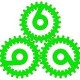We know that some participants in Harappa Ancestry Project had also submitted their data to Dodecad Project. And they were curious how the different ancestry components here lined up with the Dodecad ones.

So I decided to compare the two. I took the ancestral component percentages for the reference populations from

I selected the 36 populations that are present in both. While some of these are still not comparable because of which samples out of these populations were selected to be included in the reference datasets for Dodecad and Harappa, we are using mean values, so barring any big outliers we can compare them.

I decided to find a solution to linear equations of the form:

C1 = a11*D1 + a12*D2 + a13*D3 + a14*D4 + a15*D5 + a16*D6 + a17*D7 + a18*D8 + a19*D9 + a1A*D10
C2 = a21*D1 + a22*D2 + a23*D3 + a24*D4 + a25*D5 + a26*D6 + a27*D7 + a28*D8 + a29*D9 + a2A*D10
C3 = a31*D1 + a32*D2 + a33*D3 + a34*D4 + a35*D5 + a36*D6 + a37*D7 + a38*D8 + a39*D9 + a3A*D10
C4 = a41*D1 + a42*D2 + a43*D3 + a44*D4 + a45*D5 + a46*D6 + a47*D7 + a48*D8 + a49*D9 + a4A*D10
C5 = a51*D1 + a52*D2 + a53*D3 + a54*D4 + a55*D5 + a56*D6 + a57*D7 + a58*D8 + a59*D9 + a5A*D10
C6 = a61*D1 + a62*D2 + a63*D3 + a64*D4 + a65*D5 + a66*D6 + a67*D7 + a68*D8 + a69*D9 + a6A*D10
C7 = a71*D1 + a72*D2 + a73*D3 + a74*D4 + a75*D5 + a76*D6 + a77*D7 + a78*D8 + a79*D9 + a7A*D10
C8 = a81*D1 + a82*D2 + a83*D3 + a84*D4 + a85*D5 + a86*D6 + a87*D7 + a88*D8 + a89*D9 + a8A*D10
C9 = a91*D1 + a92*D2 + a93*D3 + a94*D4 + a95*D5 + a96*D6 + a97*D7 + a98*D8 + a99*D9 + a9A*D10

For each of the 36 populations, we'll have these 9 equations where C1 through C9 are the ancestral component percentages of that population in Harappa Project and D1 through D10 are the ancestral percentages in Dodecad Project.

The unknowns are the coefficients "a". They are 90 unknowns. Since we have 36 populations, the number of equations is 36*9=324. Therefore, this is an overdetermined system of linear equations and we can find a least squares solution to it.

Here is the solution:

D1 W Asian D2 NW African D3 S Euro D4 NE Asian D5 SW Asian D6 E Asian D7 N Euro D8 W African D9 E African D10 S Asian
C1 S Asian 0 0 0 0 0 0 0 0 0 0.92
C2 Kalash 0.54 0 -0.05 0.12 0.07 0 0.2 0 0 0.1
C3 SW Asian 0.46 0.56 0.44 0 0.9 0 -0.09 0 0.09 -0.07
C4 SE Asian 0 0 0 0 0 0.6 0 0 0 0
C5 Euro 0 0.19 0.6 0.05 -0.05 0 0.88 0 0 0
C6 Papuan 0 0 0 0 0 0 0 0 0 0
C7 NE Asian 0 0 0 0.85 0 0.4 0 0 0 0
C8 W African 0 0.12 0 0 0 0 0 1 0 0
C9 E African 0 0.12 0 0 0.05 0 0 0 0.89 0

Don't take the exact values to heart but this shows the general relationship between the Dodecad and Harappa (K=9) ancestral components.

The South Asian components are about the same in both projects.

The Kalash component is a mix but is primarily Dodecad West Asian.

The Harappa Southwest Asian has contributions from Northwest African, West Asian and South European in addition to the Dodecad West Asian component.

The Southeast Asian component corresponds partially to the Dodecad East Asian component.

The Harappa European component is more Dodecad North European than South European.

If enough Harappa-Dodecad participants are willing to let me know their IDs for both projects, I can do a similar analysis using individual data.

1.Dude

HRP0029/DOD387

2.razib

HRP002/DOD075

3.Paul Givargidze

HRP0010/DOD134

4.sv

HRP0016/DOD327

5.RK

HRP0017/DOD331

Were the coefficients similar across all populations?

•Zack

No. The error was highest for Harappa European and Southwest Asian components. And the errors were most cases concentrated among specific groups.

6.SA

HRP0013/DOD336

7.Zack

Thanks, guys, for the IDs. I'll do the analysis sometime in the coming week.

8.Sid

HRP0024/DOD414